﻿ 手机销售量预测数学模型

# 手机销售量预测数学模型Mathematical Model of Mobile Phone Sales Forecast

Abstract: Forecasting the sales volume and making a good sales volume forecast are important bases for manufacturers to make a good production plan. In this paper, the sales volume and the order volume prediction of the latest generation of mobile phone products are studied. On the basis of the data given in the topic, the author selects the appropriate scientific principles and forecasting methods, to process and mine the data, and establish a forecasting model, and then processes and analyzes the model, studies and makes a forecasting model of mobile phone sales, so as to provide convenience for the sales of merchants. This paper focuses on the relevant knowledge of statistical learning theory for statistical estimation and prediction. Because the practical part of statistical learning theory is support vector machine, which can effectively realize the design method of minimizing structural risk, this paper adopts the method of least square support vector machine. Based on machine learning theory and statistical theory, a complete modeling work is carried out by using support vector machine. The prediction results show that the model has high prediction accuracy, and based on various conditions such as influencing factors of mobile phone sales, it realizes the systematic prediction of mobile phone sales, which has good development and can provide convenient conditions for electronic enterprise manufacturers.

1. 引言

2. 背景介绍

3. 模型假设与符号说明

3.1. 模型假设

1) 假设附件中所给的数据完全真实可靠。

2) 假设在已知数据时间段和预测时间段内，市场没有发生大的政策改变、销售模式改变。

3) 假设所给的电子产品没有出现较大的质量问题导致大规模产品召回。

4) 忽略电子产品退回后对订单的影响。

3.2. 符号说明

4. 模型建立与求解

4.1. 问题一分析

4.2. 模型建立与输入输出量选取

4.2.1. 建立网络模型

4.2.2. 选择输入量和输出量

4.2.3. 确定销售量预测结构模型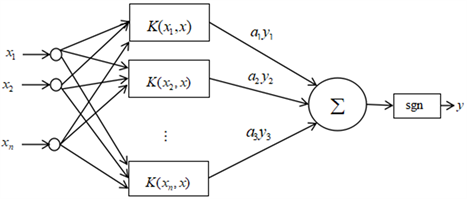Figure 1. Support vector machine model

4.2.4. 时间序列模型的定阶

4.2.5. 建立预测模型

$\left({x}_{1},{y}_{1}\right),\cdots ,\left({x}_{l},{y}_{l}\right)\in {R}^{n}×R$

$f\left(x\right)={w}^{\text{T}}\phi \left(x\right)+b$

$\begin{array}{l}\mathrm{min}\frac{1}{2}{‖w‖}^{2}+r\frac{1}{2}\left(\underset{i=1}{\overset{l}{\sum }}{\xi }_{{i}_{i}}^{2}\right)\\ \text{st}\text{\hspace{0.17em}}\left\{\begin{array}{l}{y}_{i}={w}^{\text{T}}\phi \left({x}_{i}\right)+b+{\xi }_{i}\\ {\xi }_{i}\ge 0\\ i=1,\cdots ,l\end{array}\end{array}$ (1)

$L=\frac{1}{2}{‖w‖}^{2}+r\frac{1}{2}\left(\underset{i=1}{\overset{l}{\sum }}{\xi }_{i}^{2}\right)-\underset{i=1}{\overset{l}{\sum }}{a}_{i}\left({w}^{\text{T}}\phi \left({x}_{i}\right)+b+{\xi }_{i}-{y}_{i}\right)$

$\left\{\begin{array}{l}\frac{\partial l}{\partial w}=0 \to w=\underset{i=1}{\overset{l}{\sum }}{a}_{i}\phi \left({x}_{i}\right)\\ \frac{\partial l}{\partial b}=0\to \underset{i=0}{\overset{l}{\sum }}{a}_{i}=0\\ \frac{\partial l}{\partial {\xi }_{i}}=0\to {a}_{i}=r{\xi }_{i}\\ \frac{\partial l}{\partial {\xi }_{i}^{\ast }}=0\to {w}^{\text{T}}\phi \left({x}_{i}\right)+b+{\xi }_{i}=0\end{array}$

$\left[\begin{array}{ccccc}0& 1& 1& \cdots & 1\\ 1& K\left({X}_{1},{X}_{2}\right)+1/r& K\left({X}_{1},{X}_{2}\right)& \cdots & K\left({X}_{1},{X}_{n}\right)\\ 1& K\left({X}_{2},{X}_{1}\right)& K\left({X}_{2},{X}_{2}\right)+1/r& \cdots & K\left({X}_{2},{X}_{n}\right)\\ ⋮& ⋮& ⋮& \ddots & ⋮\\ 1& K\left({X}_{n},{X}_{1}\right)& K\left({X}_{n},{X}_{2}\right)& \cdots & K\left({X}_{n},{X}_{n}\right)+1/r\end{array}\right]\left[\begin{array}{c}b\\ {a}_{1}\\ {a}_{2}\\ ⋮\\ {a}_{3}\end{array}\right]=\left[\begin{array}{c}0\\ {y}_{1}\\ {y}_{2}\\ ⋮\\ {y}_{3}\end{array}\right]$

$f\left(x\right)={w}^{\text{T}}\phi \left(x\right)+b=\underset{i=1}{\overset{l}{\sum }}{a}_{i}K\left({x}_{i},x\right)+b$ (2)

$f\left(x\right)=\underset{i=1}{\overset{l}{\sum }}{a}_{i}k\left({x}_{i},x\right)+b$ (3)

1) 对已知数据进行分析和预处理处理(特征提取、归一化)；

2) 形成训练样本集和测试样本集；

3) 针对训练样本建立目标函数；

4) 解出目标函数；

5) 将求解式所得的参数代入回归函数f(x)，将样本对未来20周手机销售量进行预测；

6) 依据SVM手机销售模型建立手机销售量预测的系统。Figure 2. Modeling process of predicting mobile phone sales

4.2.6. 数据处理

4.3. 问题一求解

a-3销售量预测，以产品a-3的销售量为因变量，以生产商收到的订单、销售商的下单量、顾客退货量为自变量，建立预测模型，模型拟合值与预测值的拟合程度如图3图4所示：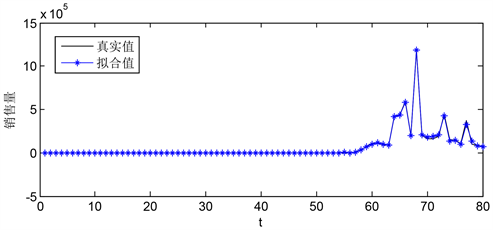Figure 3. a-3 model fitting diagram of predicted data and actual data (1~80 weeks)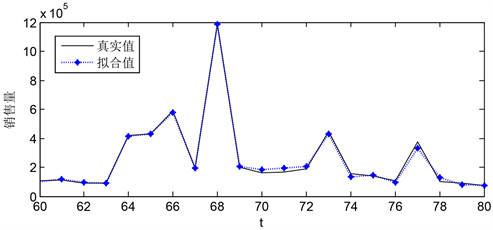Figure 4. a-3 model fitting diagram of predicted data and actual data (60~80 weeks)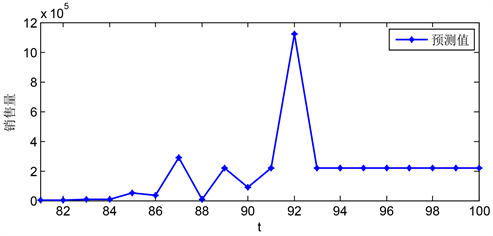Figure 5. a-3 predicted data predicted value in the next 20 weeks

b-2销售量预测，以产品b-2的销售量为因变量，以生产商收到的订单、销售商的下单量、顾客退货量为自变量，建立预测模型，模型拟合值与预测值的拟合程度如图6图7所示。图8为b-2预测数据未来20周销售量的预测值。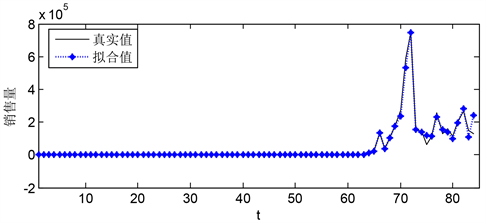Figure 6. b-2 model fitting diagram of predicted data and actual data (0~80 weeks)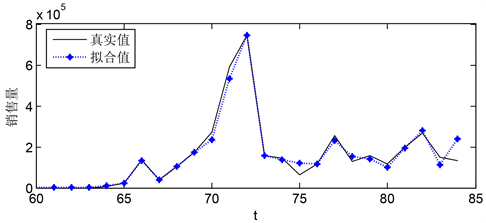Figure 7. b-2 model fitting diagram of predicted data and actual data (60~85 weeks)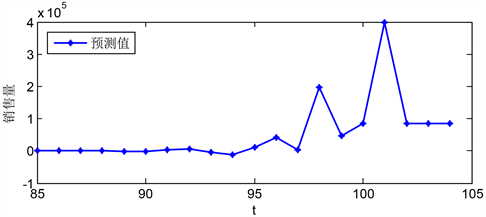Figure 8. b-2 sales forecast for the next 20 weeks

c-1销售量预测，以产品c-1的销售量为因变量，以生产商收到的订单、销售商的下单量、顾客退货量为自变量，建立预测模型，预测数据与实际数据模型拟合图(0~50周)如图9所示，图10为b-2预测数据未来20周销售量的预测值。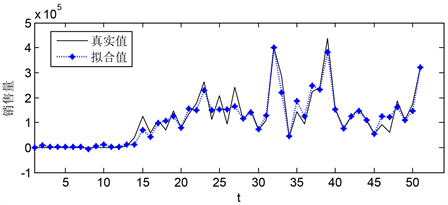Figure 9. Model fitting diagram of c-1 predicted data and actual data (0~50 weeks)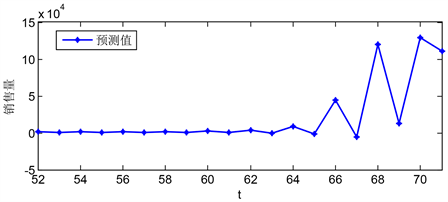Figure 10. c-1 sales forecast for the next 20 weeks

4.4. 问题二求解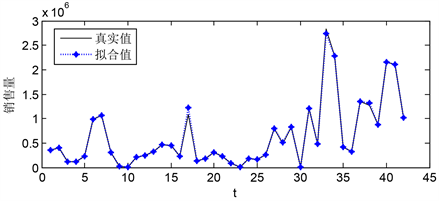Figure 11. Model fitting diagram of predicted data and actual data (0~45 weeks)Figure 12. e-2 forecast value of Class A + B orders in Area A in the next 20 weeks

2) 产品d-3在a区B + C类订单预测，按照时间序列以产品d-3的B + C类订单为输出变量，建立预测模型，模型拟合值与预测值的拟合程度如图13图14为d-3未来20周销售量的预测值。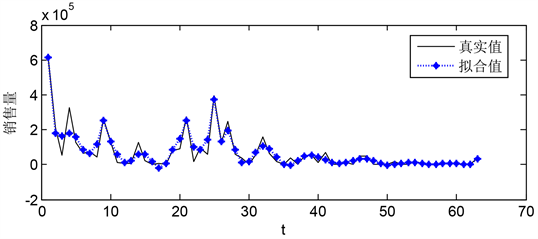Figure 13. Model fitting diagram of predicted data and actual data (0~70 weeks)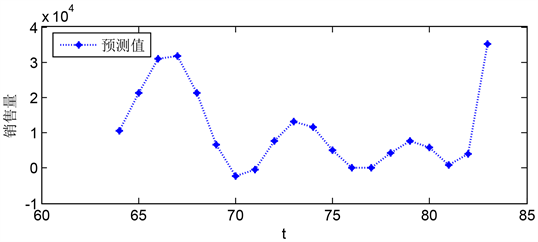Figure 14. d-3 forecast value of B + C orders in area a in the next 20 weeks

3) 产品到在a区A + B类订单预测，按照时间序列以产品d-3的A + B类订单为输出变量，建立预测模型，模型拟合值与预测值的拟合程度如图15图16为d-3预测数据未来20周销售量的预测值。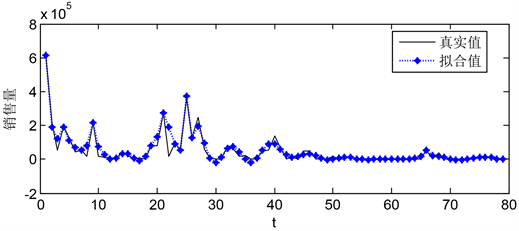Figure 15. Model fitting diagram of predicted data and actual data (0~80 weeks)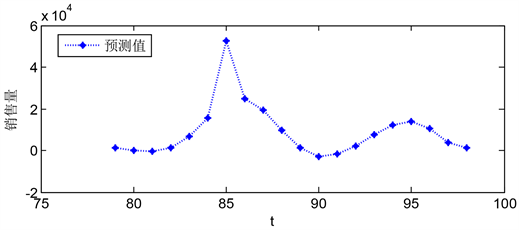Figure 16. d-3 forecast value of Class A + B orders in Area A in the next 20 weeks

5. 模型评价

5.1. 模型优点

5.2. 模型缺点

 胡俊. 基于最小二乘法支持向量机的小麦产量预测方法研究[D]: [硕士学位论文]. 西安: 西安科技大学, 2014.

 曹旭东. 数学建模原理与方法[M]. 北京: 高等教育出版社, 2014.

 刘科峰, 张韧, 于鹏, 王彦磊, 余丹丹. 基于小波分解和最小二乘支持向量机的西太平洋副高预测[J]. 热大气象学报, 2007, 12(5): 32-41.

Top